Mobile QR Code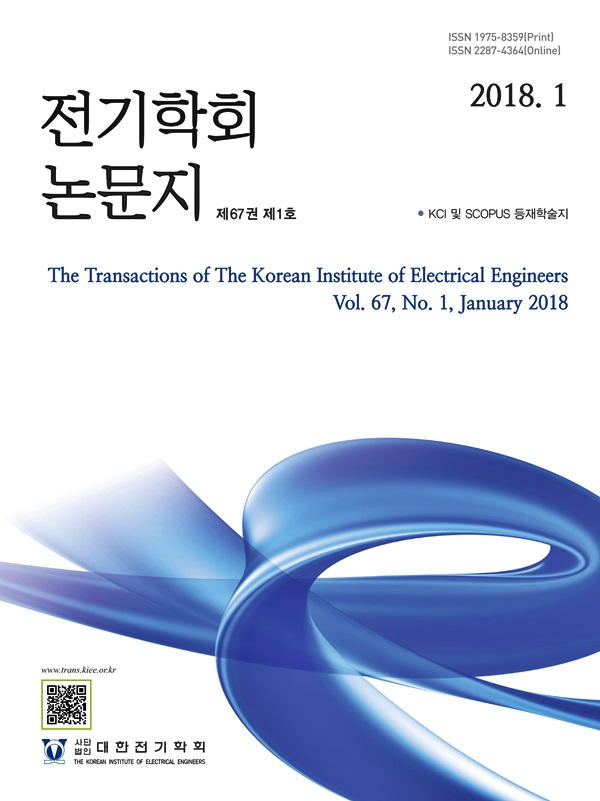#### The Transactions of the Korean Institute of Electrical Engineers

##### ISO Journal TitleTrans. Korean. Inst. Elect. Eng.

1. (School of Electrical and Electronic Engineering, Chung-Ang University, Korea.)

Three-phase four-wire, Active Thermal Control, Carrier-Based Pulse-Width-Modulation, Model Predictive Control

## 1. Introduction

The global development in industrialization increases energy consumption, resulting in the continued usage of fossil fuels. Rising carbon dioxide emissions from the combustion of fossil fuels have accelerated global warming, causing major environmental concerns. Electricity generated from renewable energy sources like photovoltaic (PV), wind, or biomass might take the place of fossil fuel-based electricity generation. The design of a grid-connected converter, as depicted in Fig. 1, is critical for such systems because it supplies stable AC power to the grid and loads from the DC power of the renewable energy source.

The increasing use of power semiconductor devices is known to cause serious problems in the electric power system. The power semiconductor devices play an important role in the power converters but are the most fragile component (1). As a result, the power semiconductor components have a significant impact on the dependability of power converters, which necessitates a high-reliability standard for the power converter. According to (2), the failures in a power semiconductor device are mostly caused by thermal stress, accounting for 60\%. ATC is a recently introduced technique for adjusting power losses and thermal stress. The basic approach is to use thermal control to influence the power losses within the power converter. The reduction of the power losses results in a decrease in the average temperature and thermal cycles. Using a classification approach based on converter types, the author in (3) examines the ATC techniques by classifying ATC according to the various converter kinds. Adjusting converter's switching frequencies are the most common and easy method for achieving thermal management, which has a direct influence on power losses without significantly affecting the power system's operational state. By reducing the operation switching frequency, the switching losses of the converter decrease, resulting in the reduction of power losses (3). A different ATC scheme is to modify the modulation methods. This strategy is usually applied in multilevel converters where the available redundant switching states reduce the switching losses or conduction losses (3). The discontinuous PWM (DPWM) is a well-known strategy whose basic concept is to clamp the voltage reference of the converter output to the upper or lower DC-link potential for a predetermined interval so that the corresponding power device maintains its status without switching, thereby mitigating switching loss in that interval. Due to the reduced power device loss during the clamping time, the junction temperature mean value and variance are also lowered. Switching frequency is typically used to influence converter junction temperature, which can have a negative impact on output performance in low-level systems. The ATC strategies using a modified modulation method can reduce the power loss of the specific stressful device but are complicated. However, these ATC approaches do not take into consideration that the phase legs of the converter can have different aging conditions. For power semiconductors, manufacturing techniques have been recognized as a possible source of failure. It leads to a typical distortion in the quality of the components, which results in different aging conditions. In order to improve the performance of the three-phase converter, phase legs are supplied with varying amounts of electricity based on their respective aging conditions. The goal is to avoid or postpone as much as possible any failures. The aging condition can be observed (4), and the power is distributed accordingly, whereby more aged phase leg is supplied less power or even no power. In this way, the power-dependent failures in the phase legs are delayed. In the case that the phase leg can just supply part power to the corresponding load, the grid will provide the rest power of the load, as shown in Fig. 1. This is equivalent to unbalanced load condition in practical situations (5). As a critical component of power distribution, the three-phase converter should be able to provide an unbalanced load in islanding mode and deliver regulated AC voltages due to this evolution of the custom power concept for each phase leg.

Fig. 1. Typical configuration of the grid-connected system.Fig. 2. Configuration circuit of the two-level three-phase four-wire converter with balance circuit.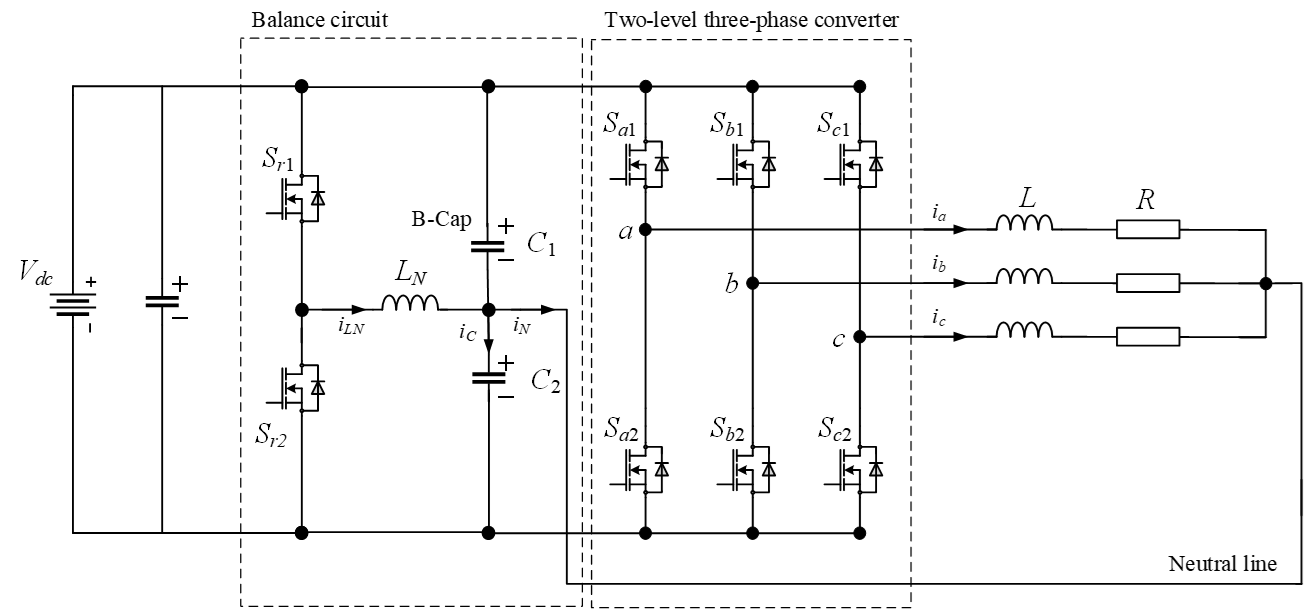The three-phase legs of the two-level three-phase four-wire converter are totally decoupled, allowing for independent control of each phase converter. This allows for the implementation of ATC through unevenly loading each phase of the converter. By changing the output power of each phase in accordance with the deterioration of power semiconductor components, the lifetime of converter can be prolonged, as indicated earlier. In this paper, an ATC approach based per-phase power control in the two-level three-phase four-wire converter with balanced circuit is presented. An MPC approach for the two-level three-phase four-wire converter with balanced circuit is investigated in terms of per-phase control capability. An improved balance circuit control scheme is proposed using a modified cost function with a neutral current feed-forward term to reduce the capacitor voltage ripple. Additionally, a CBPWM-based controller using per-phase voltage and current control is also presented. Both MPC and CBPWM-based controllers are investigated in this paper regarding regulating power capability in each phase leg. This paper presents the comprehensive study of the two-level three-phase four-wire converter with balance circuit. The ATC method, including MPC and CBPWM-based controllers, is investigated to evaluate the capability of regulating phase leg output power depending on its aging condition. The simulation and experiment are conducted to confirm the operation of the ATC strategy based per-phase control in the two-level three-phase four-wire converter with balance circuit.

## 2. Description of the two-level three-phase four-wire converter with balance circuit

### 2.1 Structure and Operation

The topology of the two-level, three-phase, four-wire converter with a balance circuit examined in this study is depicted in Fig. 2. This system contains a DC source, a two-level three-phase converter, and a balance circuit. Compared with the conventional two-level three-phase converter, the neural point of load is connected to the midpoint of the DC-link capacitors. As shown in Fig. 2, the converter is supplied by a DC voltage $V_{dc}$ energized by any distributed sources, such as fuel cells, photovoltaic devices, and wind turbines. The converter outputs are connected to a three-phase $L$ filter and $R$ load. The three-phase converter line-to-neutral voltages are denoted by $v_{a}$, $v_{b}$, and $v_{c}$. The three-phase converter output currents are $i_{a}$, $i_{b}$, and $i_{c}$.

According to different switching patterns, the two-level three-phase four-wire converter has two operation statuses per phase, as in the conventional two-level three-phase converter. The voltage of the phase leg $v_{x}(x=a,\:b,\:c)$ respected to the neutral point can be obtained as where $S_{x}$ denotes the phase leg

Table 1. Available switching states and corresponding output voltage of the two-level three-phase four-wire converter.

 Voltage vectors Switching state Voltage $S_{a}$ $S_{b}$ $S_{c}$ $v_{a}$ $v_{b}$ $v_{c}$ $v_{1}$ -1 -1 -1 $-\dfrac{Vdc}{2}$ $-\dfrac{Vdc}{2}$ $-\dfrac{Vdc}{2}$ $v_{2}$ -1 -1 1 $-\dfrac{Vdc}{2}$ $-\dfrac{Vdc}{2}$ $\dfrac{Vdc}{2}$ $v_{3}$ -1 1 -1 $-\dfrac{Vdc}{2}$ $\dfrac{Vdc}{2}$ $-\dfrac{Vdc}{2}$ $v_{4}$ -1 1 1 $-\dfrac{Vdc}{2}$ $\dfrac{Vdc}{2}$ $\dfrac{Vdc}{2}$ $v_{5}$ 1 -1 -1 $\dfrac{Vdc}{2}$ $-\dfrac{Vdc}{2}$ $-\dfrac{Vdc}{2}$ $v_{6}$ 1 -1 1 $\dfrac{Vdc}{2}$ $-\dfrac{Vdc}{2}$ $\dfrac{Vdc}{2}$ $v_{7}$ 1 1 -1 $\dfrac{Vdc}{2}$ $\dfrac{Vdc}{2}$ $-\dfrac{Vdc}{2}$ $v_{8}$ 1 1 1 $\dfrac{Vdc}{2}$ $\dfrac{Vdc}{2}$ $\dfrac{Vdc}{2}$

##### (1)
$v_{x}=S_{x}\times\dfrac{V_{dc}}{2}$

switching state, i.e., $S_{x}=1$ when the upper switch is ON, and $S_{x}=-1$ when the upper switch is OFF. The three control signals $S_{a}$, $S_{b}$, $S_{c}$ form a total of 8 (23) switching states. The available switching states with the corresponding output voltage in each phase are presented in Table 1.

The balance circuit is composed of two switches ($S_{r1}$ and $S_{r2}$) and a balance circuit inductor $L_{N}$ and two split DC-link capacitors ($C_{1}$ and $C_{2}$), as shown in the dotted box of Fig. 2. The balance circuit is controlled to stabilize the capacitor voltages. $S_{r1}$ and $S_{r2}$ are managed to balance capacitor voltages and cause the neutral current to flow through the balance circuit inductor.

### 2.2 ATC for each phase leg of the two-level three-phase four-wire converter with balance circuit

As stated previously, the two-level three-phase four-wire converter has to drive both single-phase and three-phase loads. In a practical system, the three-phase load is not completely balanced too. This leads to different aging conditions in each phase leg and the lifetime of the converter. To overcome the problem of different remaining lifetimes of phase legs, ATC strategy based per-phase control is needed to extend the lifetime of the more aged phase legs. The objective is to allow the more aged phase legs work properly until the next maintenance is implement by stressing them less than the other ones. Fig. 3(a) shows the case that phase legs $b$ and $c$ are the most aged legs, meaning that two phase legs $b$ and $c$ have low remaining lifetime with respect to phase $a$. To ease the aging condition of phase leg, the corresponding output power in phases $b$ and $c$ can be decreased to reduce the power losses, as shown in Fig. 3(a). It lowers the thermal stress of the power semiconductor devices in the phase legs $b$ and $c$, resulted in increasing lifetime. Fig. 3(b) shows the case that phase $c$ is the most aged leg. The same procedure is realized by reducing the output power of phase $c$ to increase the corresponding lifetime. In Fig. 3(c), assuming that three phase legs have different aging condition, resulted in different output power.

Fig. 3. ATC strategy by adjusting the output power of phase leg (a) Phases $b$ and $c$ are the most aged leg, (b) Phase $c$ is the most aged leg, (c) Phases $a$, $b$, and $c$ have different aging conditions.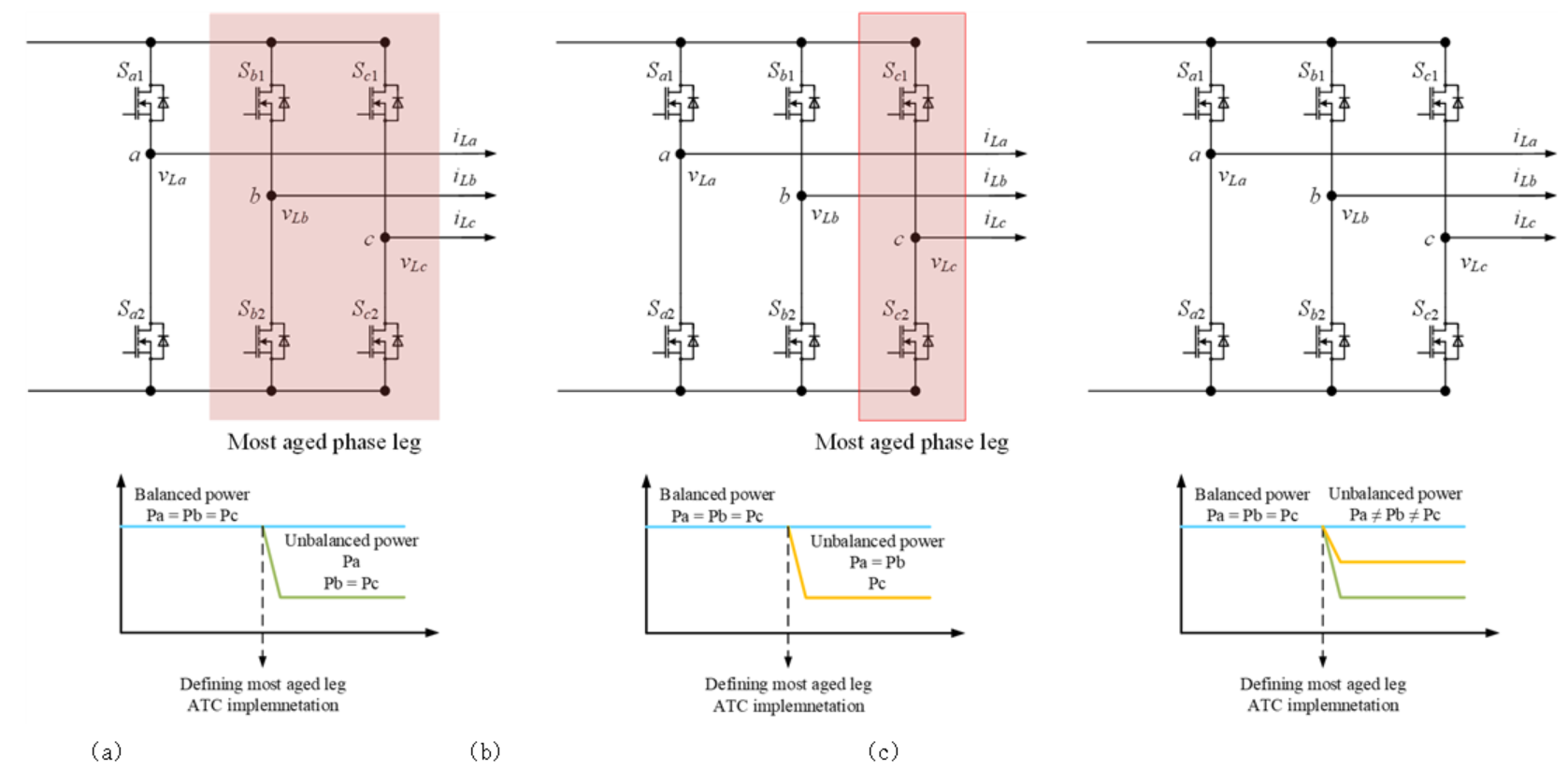## 3. MPC-based controller

The two-level three-phase four-wire converter with balance circuit, connected to $R-L$ load, shown in Fig. 2, is considered to generate the mathematical model of the converter. As indicated previously, the relationship among the switching states and load voltage is expressed in (1). The three control signals $S_{a}$, $S_{b}$, $S_{c}$ from a total of 8 (23) switching states. The valid switching states with the corresponding output voltage in each phase are presented in Table 1.

Assuming that the capacitor voltages are balanced at nominal value $V_{dc}/2$. Each phase of the two-level three-phase four-wire converter can be analyzed separately. To implement ATC by adjusting the output power in each phase leg, the MPC scheme is realized in $abc-$ frame instead of $dq-$ frame as conventional MPC. By applying the Kirchhoff voltage law to Fig. 2, the output voltages of the converter can be described corresponding to $R-L$ load and the output currents ($i_{a}$, $i_{b}$, $i_{c}$).

##### (2)
$\begin{bmatrix}v_{a}\\v_{b}\\v_{c}\end{bmatrix}=R\times\begin{bmatrix}i_{a}\\i_{b}\\i_{c}\end{bmatrix}+L\times\dfrac{d}{dt}\begin{bmatrix}i_{a}\\i_{b}\\i_{c}\end{bmatrix}$

The continuous-time expression for the output current can be derived from(2) as

##### (3)
$\dfrac{d}{dt}\begin{bmatrix}i_{a}\\i_{b}\\i_{c}\end{bmatrix}=\dfrac{1}{L}\times\left\{\begin{bmatrix}v_{a}\\v_{b}\\v_{c}\end{bmatrix}-R\times\begin{bmatrix}i_{a}\\i_{b}\\i_{c}\end{bmatrix}\right\}$

Based on this, eq. (3) can be expressed in a discrete-time model by predicting the next sampling instant of output current following

##### (4)
$\begin{bmatrix}i_{a}(k+1)\\i_{b}(k+1)\\i_{c}(k+1)\end{bmatrix}=\begin{bmatrix}i_{a}(k)\\i_{b}(k)\\i_{c}(k)\end{bmatrix}+\dfrac{T_{sp}}{L}\times\left\{\begin{bmatrix}v_{a}(k)\\v_{b}(k)\\v_{c}(k)\end{bmatrix}-R\times\begin{bmatrix}i_{a}(k)\\i_{b}(k)\\i_{c}(k)\end{bmatrix}\right\}$

As shown in (4), the predicted output current in $k+1$ sampling instant requires the output current and output voltage in $k$th instant. The output voltage prediction is generated using the 8 switching states and the DC-link voltage, as indicated in (1).

In the final stage, the predicted output currents in $i_{x}(k+1)(x=a,\:b,\:c)$ are compared to the corresponding reference $i_{a}^{*}(k+1)(x=a,\:b,\:c)$, in a natural reference frame making use of cost function $g_{1}$ as follows:

##### (5)
\begin{align*} g_{1}=\left | i_{a}^{*}(k+1)-i_{a}(k+1)\right | +\left | i_{b}^{*}(k+1)-i_{b}(k+1)\right |\\ +\left | i_{c}^{*}(k+1)-i_{c}(k+1)\right | \end{align*}

The optimal switching state is one that minimizes the cost function $g_{1}$. The cost function $g_{1}$ includes three terms regarding three phases output currents. Different current references can be used to regulate the output power in the corresponding phase leg. It is the same as in indicated per-phase power current control method, the current reference for the most aged phase leg can be even reduced to zero to decrease the power losses. The reliability and lifetime of the converter will be improved.

Fig. 4. Structure of MPC of the two-level three-phase four-wire converter with balance circuit.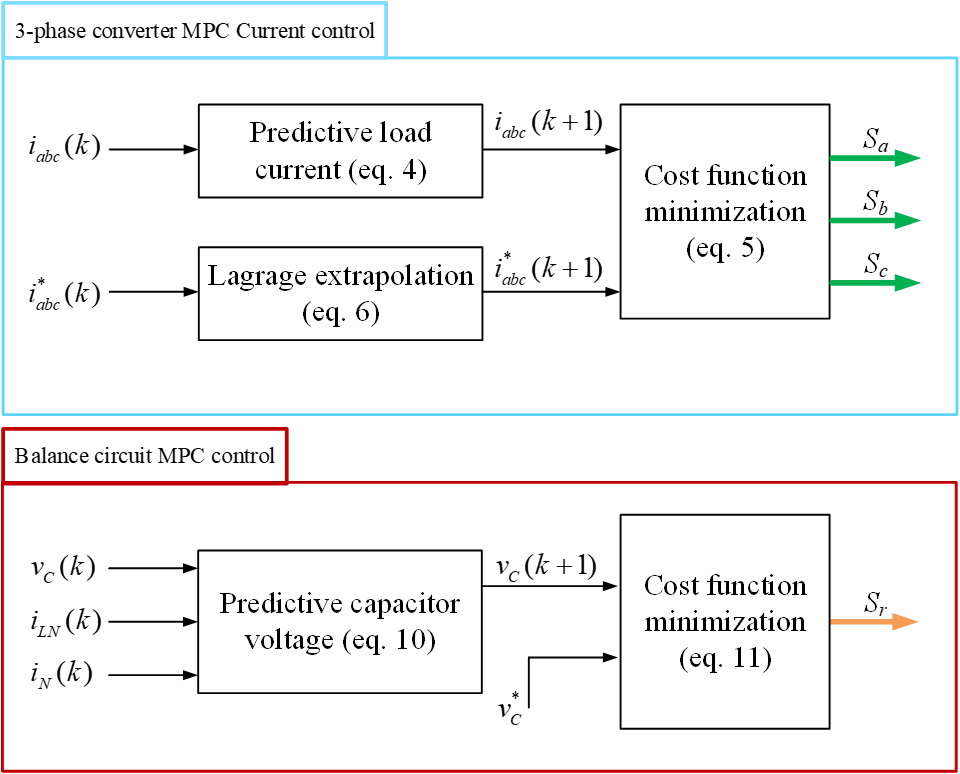The $k+1$ sampling instant of the output current references, is obtained by making use of the fourth-order Lagrange extrapolation formula as:

##### (6)
\begin{align*} \begin{bmatrix}i_{a}^{*}(k+1)\\i_{b}^{*}(k+1)\\i_{c}^{*}(k+1)\end{bmatrix}=4\begin{bmatrix}i_{a}^{*}(k)\\i_{b}^{*}(k)\\i_{c}^{*}(k)\end{bmatrix}-6\begin{bmatrix}i_{a}^{*}(k-1)\\i_{b}^{*}(k-1)\\i_{c}^{*}(k-1)\end{bmatrix}\\ +4\begin{bmatrix}i_{a}^{*}(k-2)\\i_{b}^{*}(k-2)\\i_{c}^{*}(k-2)\end{bmatrix}-\begin{bmatrix}i_{a}^{*}(k-3)\\i_{b}^{*}(k-3)\\i_{c}^{*}(k-3)\end{bmatrix} \end{align*}

The balance circuit can be described using two differential equations. One is the balance circuit inductor current dynamics, and the other is capacitor voltage dynamics. They are expressed as follows:

##### (7)
$L_{N}\dfrac{di_{\ln}}{dt}=v_{N}-v_{C2}$

##### (8)
$C_{1}\dfrac{dv_{C1}}{dt}-C_{2}\dfrac{dv_{C2}}{dt}=i_{C}=i_{\ln}-i_{N}$

where $L_{N}$ is the balance circuit inductance, $C_{1}$ and $C_{1}$ are the upper and lower DC-link capacitors, respectively. The balance circuit inductor current $i_{\ln}$, the capacitor voltages $v_{C1}$ and $v_{C2}$, the capacitor current $i_{C}$, and neutral current $i_{N}$ correspond to variables defined in Fig. 2. The neutral voltage $v_{N}$ respected to negative DC-link can be obtained as $v_{N}=V_{dc}\times S_{r}$, where $S_{r}$ denotes the balance circuit switching states, i.e., $S_{r}=1$ when the upper switch is ON, and $S_{r}=0$ when the upper switch is off. Considering the constraint of DC-link voltage $v_{C1}+v_{C2}=V_{dc}$, $i_{\ln}$ and $v_{C2}$ are selected as the state variables. Equations (7) and (8) can be expressed as:

##### (9)
$\dfrac{d}{dt}\begin{bmatrix}i_{\ln}\\v_{C2}\end{bmatrix}=\begin{bmatrix}0&\dfrac{-1}{L_{N}}\\\dfrac{1}{C_{1}+C_{2}}&0\end{bmatrix}\times\begin{bmatrix}i_{\ln}\\v_{C2}\end{bmatrix}+\begin{bmatrix}\dfrac{-1}{L_{N}}&0\\0&\dfrac{-1}{C_{1}+C_{2}}\end{bmatrix}\times\begin{bmatrix}v_{N}\\i_{N}\end{bmatrix}$

The discrete-time equations of the balance circuit inductor current and lower capacitor voltage can be achieved from (9), as demonstrated below:

##### (10)
\begin{align*} \begin{bmatrix}i_{\ln}(k+1)\\v_{C2}(k+1)\end{bmatrix}=\begin{bmatrix}i_{\ln}(k)\\v_{C2}(k)\end{bmatrix}+\begin{bmatrix}0&\dfrac{T_{sp}}{L_{N}}\\\dfrac{-T_{sp}}{C_{1}+C_{2}}&0\end{bmatrix}\times\begin{bmatrix}i_{\ln}(k)\\v_{C2}(k)\end{bmatrix}\\ +\begin{bmatrix}\dfrac{T_{sp}}{L_{N}}&0\\0&\dfrac{-T_{sp}}{C_{1}+C_{2}}\end{bmatrix}\times\begin{bmatrix}v_{N}(k)\\i_{N}(k)\end{bmatrix} \end{align*}

It should be noted that the goal of controlling balance circuit is to maintain the upper and lower capacitor voltage at nominal value. The lower capacitor voltage $v_{C2}$ is compared with the corresponding reference $v_{C}^{*}=V_{dc}/2$, using the cost function

Fig. 5. Structure of MPC for balance circuit control with neutral current feed-forward term.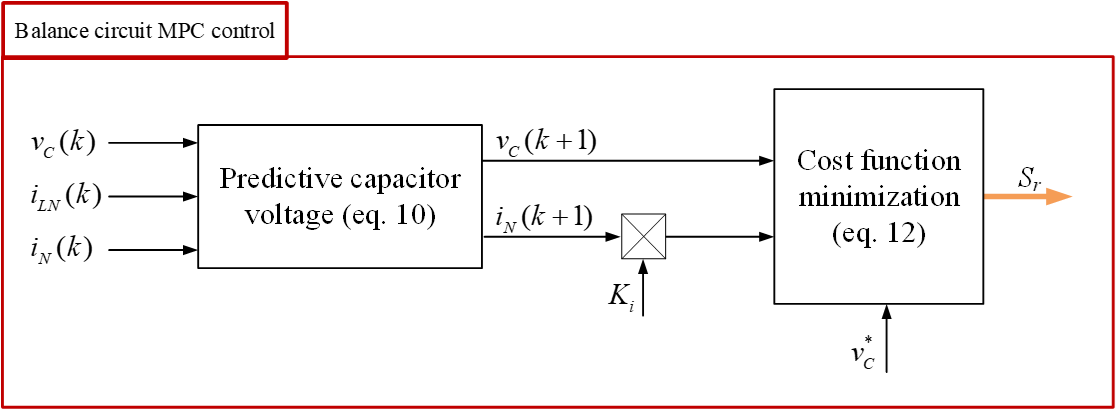##### (11)
$g_{2}=\left | v_{C}^{*}(k+1)-v_{C2}(k+1)\right |$

The MPC scheme of the two-level three-phase four-wire converter with balance circuit is depicted in Fig. 4.

Regarding balance circuit control, it is noticed that the neutral current $i_{N}$ impacts on the capacitor voltages. Consequently, a neutral current feed-forward term is presented and added to the cost function in (14) to form a modified cost function as follows:

##### (12)
$g_{2_{-}\bmod}=\left | v_{C}^{*}(k+1)+K_{i}\times i_{N}-v_{C2}(k+1)\right |$

where $K_{i}$ is the gain of the neutral current feed-forward term. Different value of $K_{i}$ will be to evaluate the effect of neutral current feed-forward term on the capacitor voltage ripple. The structure of MPC for balance circuit control with neutral current feed-forward term is depicted in Fig. 5.

## 4. CBPWM-based controller

### 4.1 Three-phase converter using per-phase voltage current control

The two-level three-phase four-wire converter can be treated as three equivalent single-phase half-bridge circuits, and a

Table 2. Parameters of the two-level three-phase four-wire converter with balance circuit in simulation.

 Parameters Simulation DC-link voltage $V_{dc}$ (V) 720 Balance circuit inductor (H) 2.7m Split DC-link capacitor (F) 50u Load inductor (H) 10m Load resistor (Ω) 15 Sampling frequency (kHz) 50

Fig. 7. Structure of balance circuit control (a) Voltage control, (b) Voltage current control.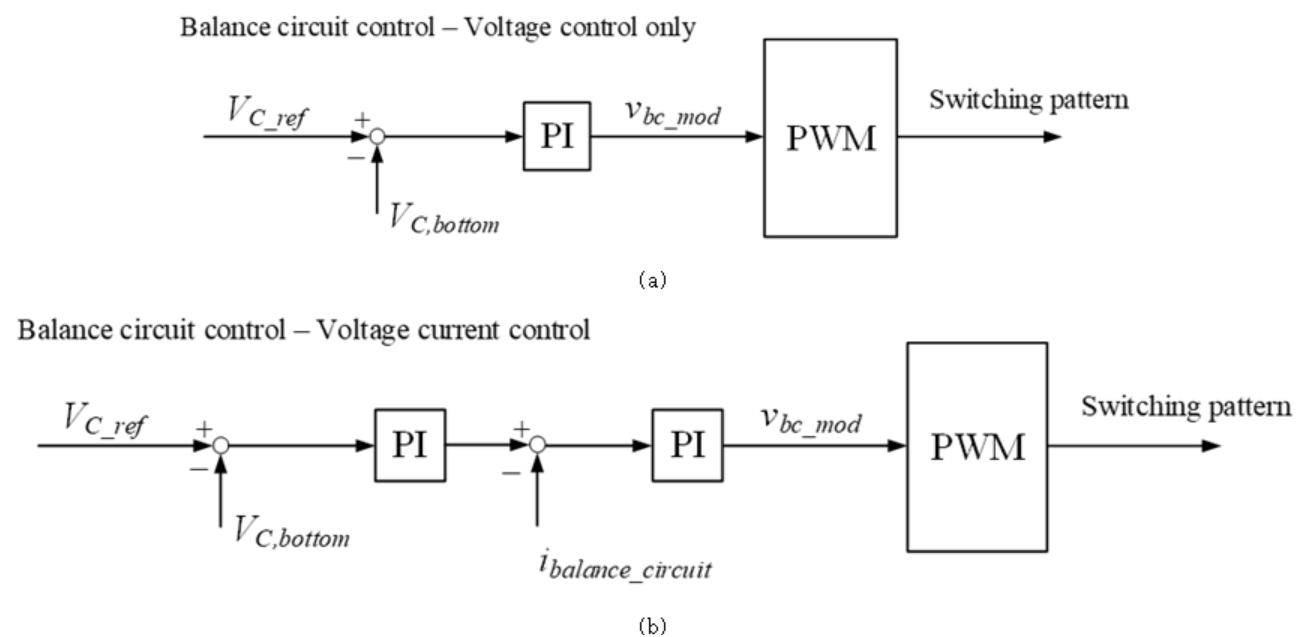per-phase control approach can be straightly employed. The per-phase control offers flexible power control to allow resilience to variable power demand. Additionally, the output power in each phase can be adequately adjusted to extend the lifetime of each phase leg based on the aging condition. In this study, a per-phase voltage current control for the two-level three-phase four-wire converter is illustrated in Fig. 6, where the generic $x$-th phase, $x=a,\:b,\:c$, is presented. The control scheme includes two parts: voltage and current based PI controllers. The error signal between measured voltage and voltage reference is fed into a modified PI controller to generate the corresponding phase current reference. The modified PI controller is produced by multiplying the integral part by the sign and the unity of corresponding phase voltage reference, as shown in Fig. 6. The output current control is achieved with a modified PI controller to generate the switching pattern for each phase, as shown in Fig. 6.

### 4.2 Balance circuit control

As stated earlier, the control of capacitor voltages is decoupled from the control of the three-phase converter. Maintaining the capacitor voltages balance at a nominal voltage value $V_{dc}/2$ is the crucial task, especially in unbalanced load conditions or different output powers for ATC implementation. A PI controller controls the balance circuit to generate a PWM pattern for $S_{r1}$ and $S_{r2}$. As for voltage control only, shown in Fig. 7(a), the voltage error between the reference capacitor voltage and the lower capacitor voltage sensed signal is regulated by using a PI controller to keep the capacitor voltages balanced generate the switching pattern for the balance circuit switches. In voltage and current control for balance circuit, shown in Fig. 7(b), the output of the voltage control part is used as a reference for the current control part. The sensed signal of balance circuit current is controlled using a PI controller and generates a switching pattern for the balance circuit switches.

## 5. Verification results

The simulation results verify the MPC scheme for the two-level three-phase four-wire converter with balance circuit is presented in this section. As depicted in the previous section, the two-level three-phase four-wire converter with balance circuit is connected to $R-L$ load, as shown in Fig. 2, which is modeled in PSIM software. The MPC is implemented following the given structure in Fig. 4. The converter system is characterized by the following parameters listed in Table 2.

Fig. 8. Simulation waveforms of output currents, capacitor voltages and neutral current using MPC in unbalanced operation. (a) Pa = Pb = 3.3kW, Pc = 1kW, (b) Pa = 3.3kW, Pb = Pc = 1kW, (c) Pa = 3.3kW, Pb = 2kW, Pc = 1kW.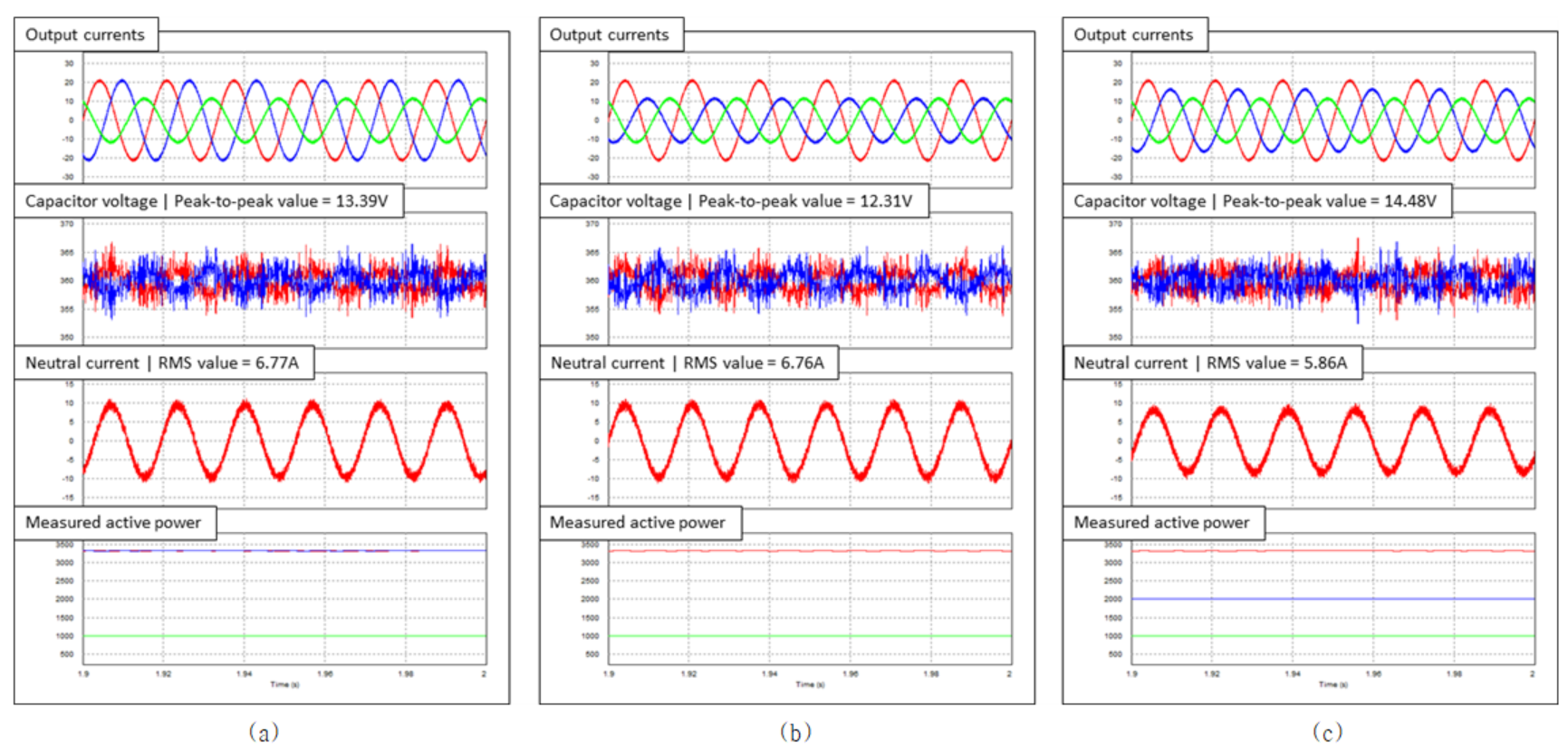In this simulation, three unbalanced operation cases are considered regarding ATC for each converter phase leg. The simulation results of the two-level three-phase four-wire converter with balance circuit using MPC under unbalanced operation where the output power in each phase is different, are presented in Fig. 8. In the first case, assuming that phase legs $c$ is the most aged, the output power of that phase $c$ should be decreased to reduce the power losses and extend the corresponding lifetime. Fig. 8(a) shows the waveforms of output currents, capacitor voltages, neutral current, and measured output power in the first case. In this case, the output power in phase $a$ and $b$ is kept the same at 3.3kW, whereas the output power in phase $c$ is reduced to 1kW. The output current waveforms are sinusoidal with the correct magnitude and phase following the output power. The capacitor voltages are kept balanced at the nominal value $V_{dc}/2$. The simulation waveforms of the second case, where phase $b$ and $c$ are the most aged, is presented in Fig. 8(b). In this case, the output power in phase $b$ and $c$ is reduced to 1kW, whereas the output power in phase $a$ is 3.3kW. As can be observed. the output currents are sinusoidal with the correct magnitude and phase following the output power. The capacitor voltages are kept balanced at the nominal value $V_{dc}/2$. In the third case, assuming that three phase legs have different aging conditions, resulted in different output power as shown in Fig. 8(c). Apparently, the output currents are sinusoidal with the correct magnitude and phase following the output power where Pa = 3.3kW, Pb = 2kW, and Pc = 1kW.

Fig. 9. Simulation waveforms of output currents, capacitor voltages and neutral current using MPC with modified cost function of balance circuit control $K_{i}=-0.2$ in unbalanced operation. (a) Pa = Pb = 3.3kW, Pc = 1kW, (b) Pa = 3.3kW, Pb = Pc = 1kW, (c) Pa = 3.3kW, Pb = 2kW, Pc = 1kW.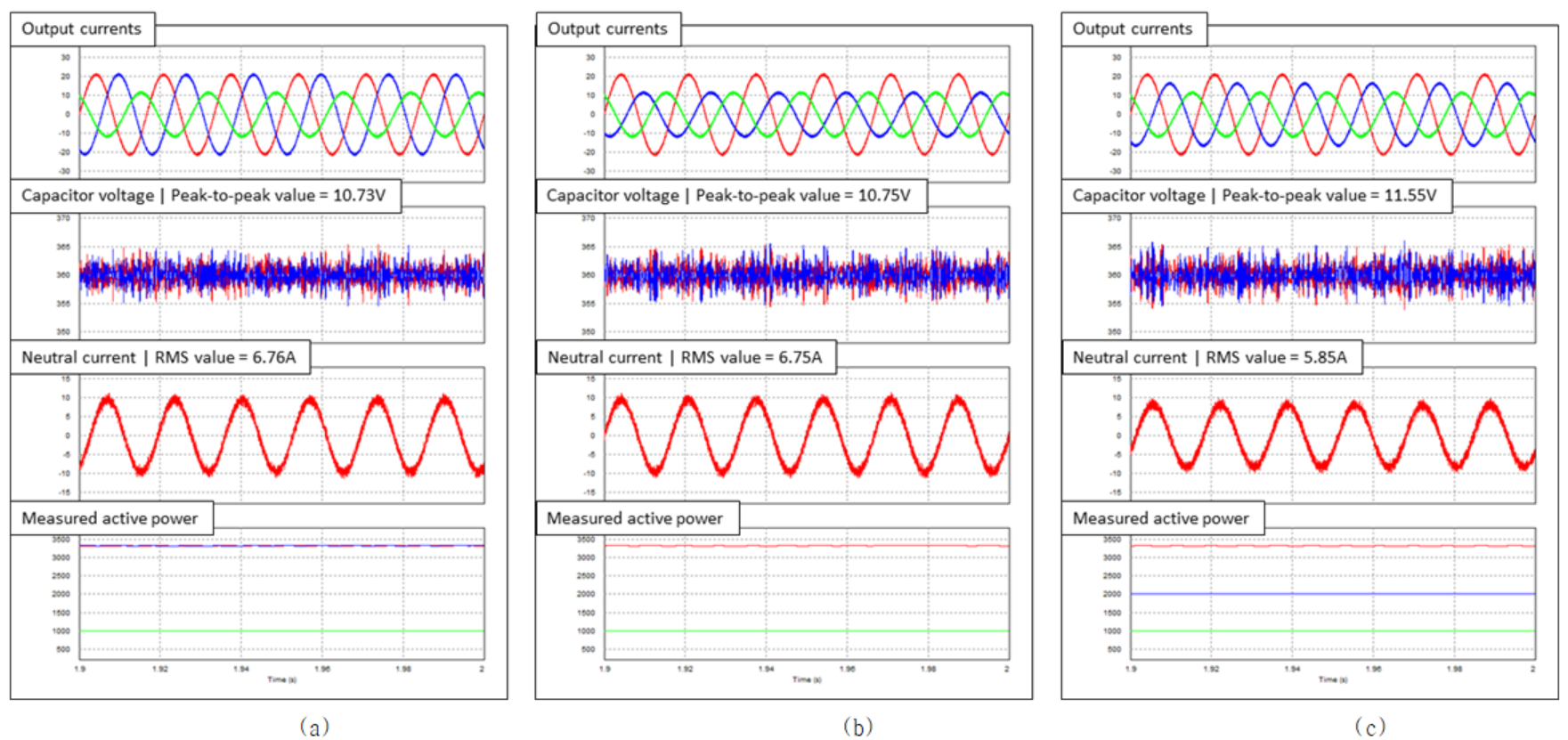Fig. 10. Simulation waveforms of output currents, capacitor voltages and neutral current using CBPWM based voltage current control in unbalanced operation. (a) Pa = Pb = 3.3kW, Pc = 1kW, (b) Pa = 3.3kW, Pb = Pc = 1kW, (c) Pa = 3.3kW, Pb = 2kW, Pc = 1kW.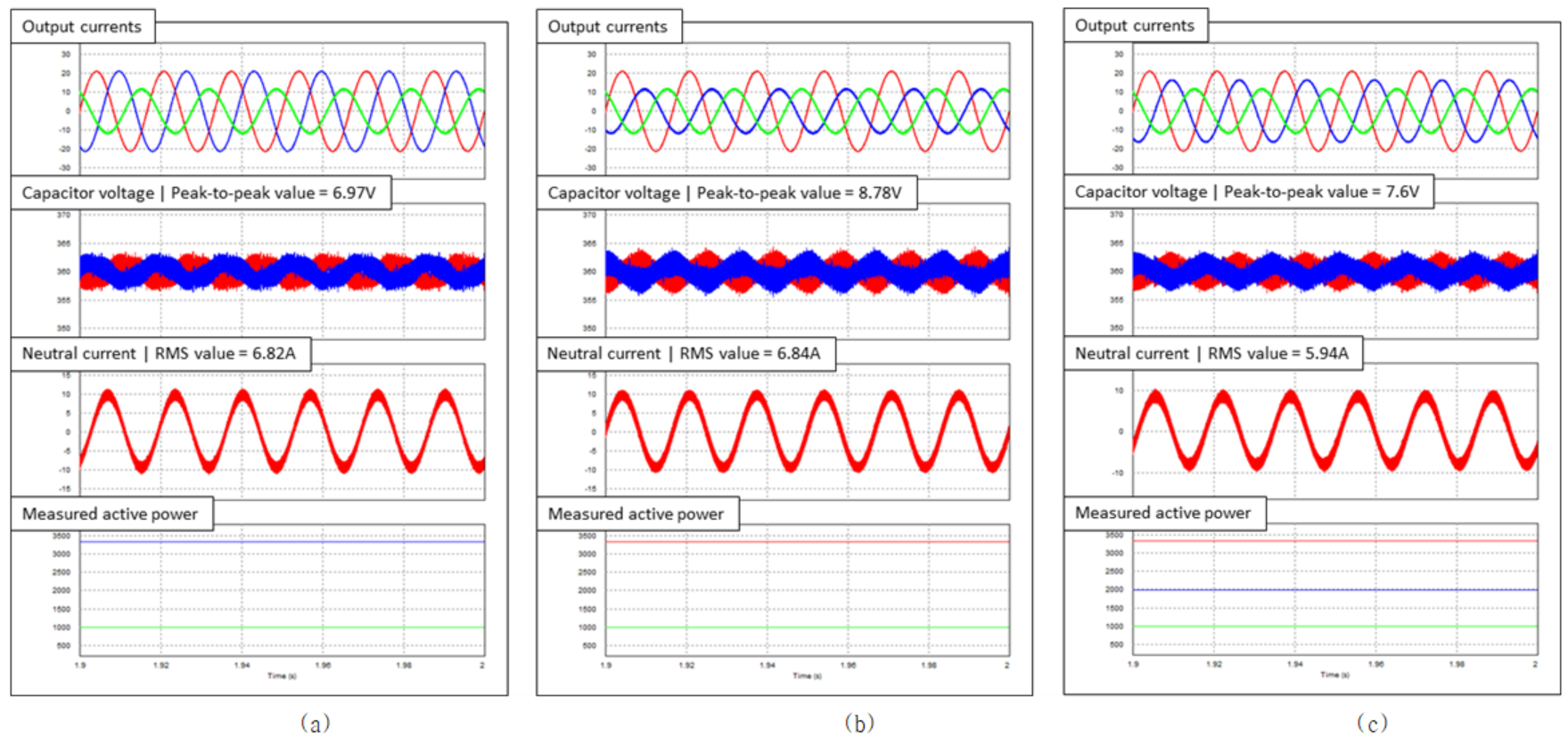Fig. 11. Experimental waveforms of output current and capacitor voltage using CBPWM based voltage current control in unbalanced operation. (a) Pa = Pb = 500W, Pc = 250W, (b) Pa = Pb = 250W, Pc = 500W, (c) Pa = 500W, Pb = 300W, Pc = 50W.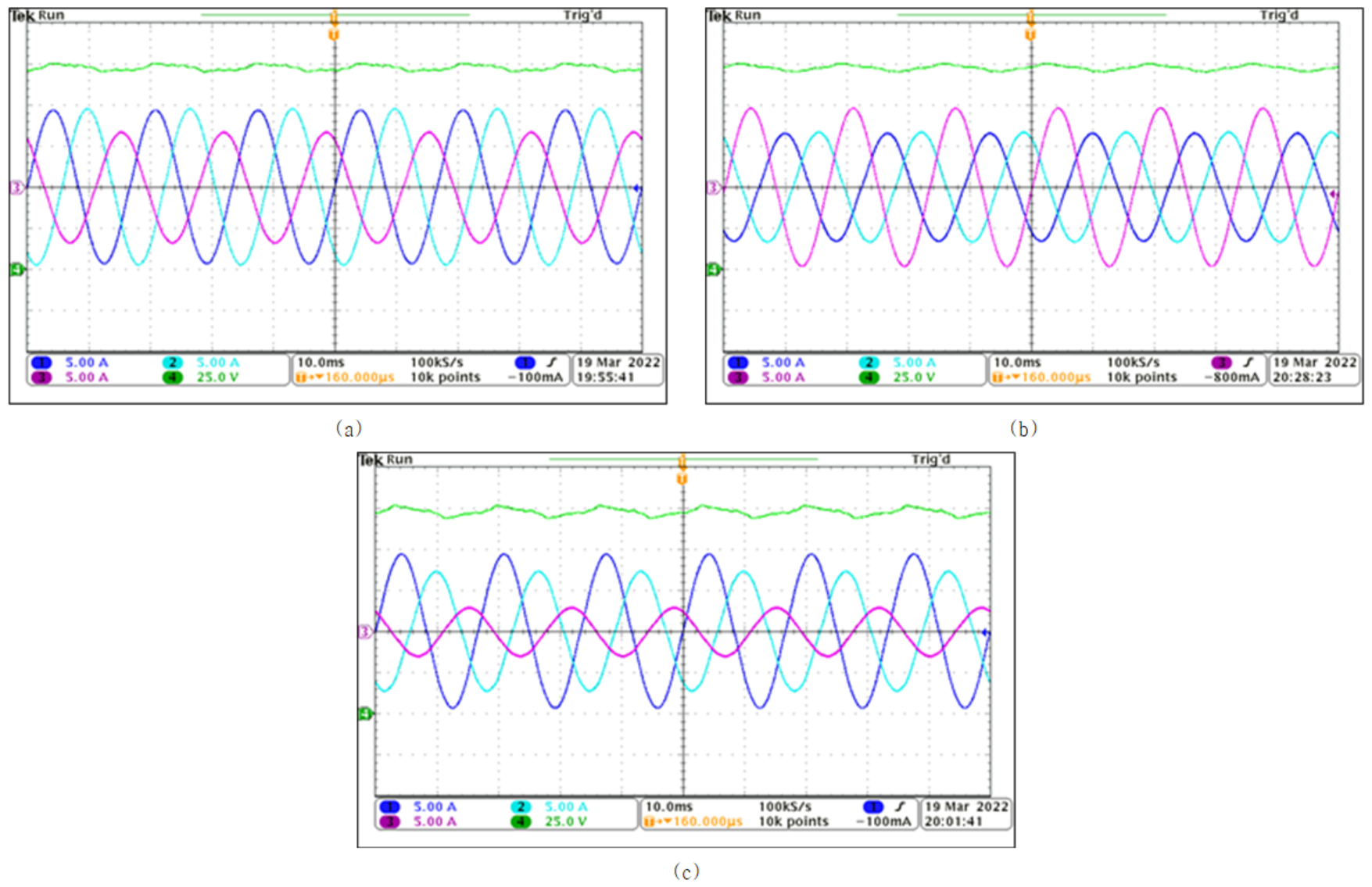As shown in (12), the modified cost function is used to decrease the capacitor voltage peak-to-peak value, as indicated earlier. Fig. 9(a) - (c) show the simulation results using a modified cost function with the gain $K_{i}=-0.2$. As can be observed, the output currents are the same as using the conventional cost function in (11). It can be seen that the capacitor voltage peak-to-peak value is reduced by about 20\% in both three cases of unbalanced operation. Meanwhile, the output currents are sinusoidal with correct phase and magnitude as using the conventional cost function. Therefore, the modified cost function of balance circuit control reduces the capacitor voltage ripple without deteriorating the converter performance.

The simulation results show that the ATC approach based MPC scheme can solve the unbalanced operation and extend the lifetime of a specific phase leg by adjusting the corresponding output power. The two-level three-phase four-wire converter with balance circuit operates correctly in both three cases of unbalanced operation.

The simulation results of the two-level three-phase four-wire converter with balance circuit using CBPWM based per-phase voltage current control under unbalanced operation where the output power in each phase is different, are presented in Fig. 10(a) – (c). Similar to the MPC scheme, in both three cases of unbalanced operation, the output currents are sinusoidal with correct phase and magnitude corresponding to the output power.

The experiment is conducted using a down-scaled prototype of the two-level three-phase four-wire converter with balance circuit. Fig. 11(a) – (c) shows the experimental waveforms of output currents and lower capacitor voltage in different cases of output power. As can be observed, the output currents are sinusoidal with correct magnitude and phase following the output power. The capacitor voltage is maintained at the nominal value $V_{dc}/2$.

The simulation and experimental results show that the per-phase power current control scheme can solve the unbalanced operation and serve as an ATC strategy to extend the lifetime of a specific phase leg by adjusting the corresponding output power.

## 6. Conclusion

This paper presents the ATC approach to regulate the output power in each phase leg depending on the aging condition for the two-level three-phase four-wire converter with balance circuit. The output power in each phase is controlled to reduce the stress for the most aged phase leg. The MPC strategy is for the two-level three-phase four-wire converter with balance circuit. The three-phase converter cost function includes three separate current control terms, which allows independently regulating the output power in each phase by changing the current reference. This contributes to increasing the lifetime of desired phase leg by adjusting the corresponding power losses. Using the neutral current feed-forward term, the modified cost function is proposed to improve the capacitor voltage control capability by reducing the capacitor voltage ripple without deteriorating the converter performance. The CBPWM based per-phase voltage current control is investigated in terms of regulating output power in each phase depending on the aging condition. The simulation and experiment results show that ATC approaches based MPC and CBPWM operated correctly in unbalanced operation.

### Acknowledgements

이 논문은 정부(과학기술정보통신부)의 재원으로 한국연구재단의 지원을 받아 수행된 연구임 (2020R1A2C1013413).

### References

1
S. Yang, A. Bryant, P. Mawby, D. Xiang, L. Ran, P. Tavner, May 2011, An Industry-Based Survey of Reliability in Power Electronic Converters, in IEEE Transaction on Industrial Application, Vol. 47, No. 3, pp. 1441-14512
L. Gopi Reddy, L. Tolbert, B. Ozpineci, May 2015, Power Cycle Testing of Power Switches: A Literature Survey, in IEEE Transaction on Power Electronics, Vol. 30, No. 5, pp. 2465-24733
M. H. Nguyen, S. Kwak, Dec 2020, Enhance Reliability of Semiconductor Devices in Power Converters, in Electronics, Vol. 9, No. 12, pp. 20684
S. Yang, D. Xiang, A. Bryant, P. Mawby, L. Ran, P. Tavner, Nov 2010, Condition Monitoring for Device Reliability in Power Electronic Converters: A Review, in IEEE Transaction on Power Electronics, Vol. 25, No. 11, pp. 2734-27525
G. Carrasco, C. A. Silva, R. Pena, R. Cardenas, Jul 2015, Control of a Four-Leg Converter for the Operation of a DFIG Feeding Stand-Alone Unbalanced Loads, in IEEE Transaction on Industrial Electronics, Vol. 62, No. 7, pp. 4630-46406
Z. Liu, J. Liu, J. Li, May 2013, Modeling, Analysis, and Mitigation of Load Neutral Point Voltage for Three-Phase Four-Leg Inverter, in IEEE Transaction on Industrial Electronics, Vol. 60, No. 5, pp. 2010-20217
P. Verdelho, G. D. Marques, Oct 1998, Four-wire current-regulated PWM voltage converter, in IEEE Transaction on Industrial Electronics, Vol. 45, No. 5, pp. 761-7708
A. Mohd, 2010, Control strategy and space vector modulation for three-leg four-wire voltage source inverters under unbalanced load conditions, in IET Power Electronics, Vol. 3, No. 3, pp. 3239
J. Liang, T. C. Green, C. Feng, G. Weiss, 2009, Increasing Voltage Utilization in Split-Link, Four-Wire Inverters, in IEEE Transaction on Power Electronics, Vol. 24, No. 6, pp. 1562-156910
T. Kerekes, R. Teodorescu, M. Liserre, C. Klumpner, M. Sumner, Sep 2009, Evaluation of Three-Phase Transformerless Photovoltaic Inverter Topologies, in IEEE Transaction on Power Electronics, Vol. 24, No. 9, pp. 2202-221111
W. Zhao, X. Ruan, D. Yang, X. Chen, L. Jia, Apr 2017, Neutral Point Voltage Ripple Suppression for a Three-Phase Four-Wire Inverter With an Independently Controlled Neutral Module, in IEEE Transaction on Industrial Electronics, Vol. 64, No. 4, pp. 2608-2619## 저자소개

##### 응웬 민 호앙(Minh Hoang Nguyen)Minh Hoang Nguyen received the B.S. degree in electrical and electronics engineering from Hanoi University of Science and Technology, Vietnam, in 2016.

He is currently pursuing the M.S and PhD combined degree in electrical and electronics engineering with Chung-Ang University, Seoul, South Korea.

His research interests are control for multilevel converters.

Sang-Shin Kwak received the Ph.D. degree in electrical engineering from Texas A&M University, College Station, TX, USA, in 2005.

From 1999 to 2000, he was a Research Engineer with LG Electronics, Changwon, South Korea.

From 2005 to 2007, he was a Senior Engineer with Samsung SDI R&D Center, Yongin, South Korea.

From 2007 to 2010, he was an Assistant Professor with Daegu University, Gyeongsan, South Korea.

Since 2010, he has been with Chung-Ang University, Seoul, South Korea, where he is currently a Professor.

His current research interests include the design, modeling, control, and analysis of power converters for electric vehicles and renewable energy systems as well as the prognosis and fault tolerant control of power electronics systems.